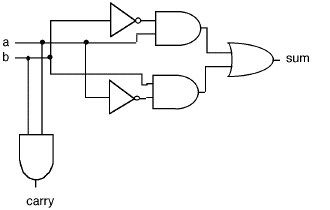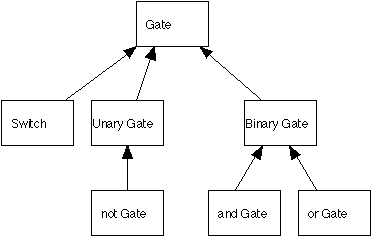# Logic Gates and Circuits

## A Java CS1 course module featuring:inheritance

Inheritance
Logic Gates
Logic Circuits

Circuit diagrams and Boolean Logic

### Introduction

This module gives a good use of inheritance in Java and also gives some needed breadth to the course as it intoduces logic gates in a way that students can actually calculate with them and connect them together into circuits.

### Student Handouts

Here is a library of sources for this project. This should be given to the students a day or so before it will be discussed in class. A simple handout on boolean logic and simple gates and circuits with standard pictures of and, or, and not gates would be useful. (Not provided here at this time.)

### Lecture Outline

2. Discussion of inheritance
3. Discussion of Boolean logic and truth tables
4. Discussion of logic gates
5. Discussion of logic circuits.A simple 1 bit half adder built with and, or, and not gates.

### In Class Exercise (team)

In small teams, the students can be asked to trace the operation of a simple circuit such as a half adder. They should also be asked to comment on why the class design did not implement a binary gate as a subclass of unary gate. Student can also be given a simple circuit diagram and then they are asked to implement this circut and test it with various inputs, recording the outputs. Another exercise is to have the teams develop the truth table for the above circuit. There are two input columns (with four combinations) and two outputs.

### Take Home Exercise (team or individual)

This exercise will have the students extend the given hierarchy. Students are askedto build nand, nor, and xor gates, similar to the and and or gates. The instructor should provide motivation for this. Students can then be asked to build an adder equivalent to the one given with and and or gates, but using nand and nor gates instead, to see the relative efficiency. This can be done with two gates. The truth table shows this clearly.

Once the students are comfortable connecting gates to get circuits, they can be asked to build a subclass of Circuit to encapsulate one of their circuit designs. See the Adder class for an example.

### Pedagogical Patterns

This exercise adds breadth to the CS1 course as well as having the students work on a reasonable sized hierarchy. This is a good example of a toybox.

### Class Diagram### Other Useful Information

Tannenbaum's Structured Computer Organization provides some background to the instructor for this module. He includes several circuit diagrams that can be used with this toolkit.